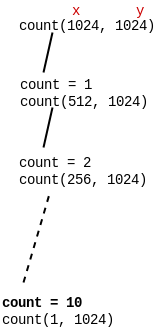Related Articles

# GATE | GATE CS 2018 | Question 44

• Last Updated : 09 Mar, 2018

Consider the following program written in pseudo-code. Assume that x and y are integers.

```Count (x, y) {
if (y !=1 ) {
if (x !=1) {
print("*");
Count (x/2, y);
}
else {
y=y-1;
Count (1024, y);
}
}
}
```

The number of times that the `print` statement is executed by the call `Count(1024, 1024)` is _______ .

Note –This was Numerical Type question.
(A) 10230
(B) 10
(C) 1023
(D) 23010

Explanation: Firstly, Count(1024, 1024) will be called and value of x will be deducted by x/2. ‘x’ will be printed 10 times. On count=10, value of x will become 1.Since x=1, inner else loop will start executing. On each call value of y will be decreased by 1 and will be executed until value of y becomes 1 (which is on 1023th call).

Since count() is called recursively for every y=1023 and count() is called 10times for each y. Therefore, 1023 x 10 = 10230